# Group Xbar-R Chart Example

Check uniformity of multiple key characteristics on a single chart.

## How Do You Use Group Xbar-R Charts?

Group Xbar and range (Xbar-R) charts help you assess changes in averages and the range of averages across measurement subgroups for a characteristic. Review the following example—an excerpt from Innovative Control Charting1—to get a sense of how a group Xbar-R chart works.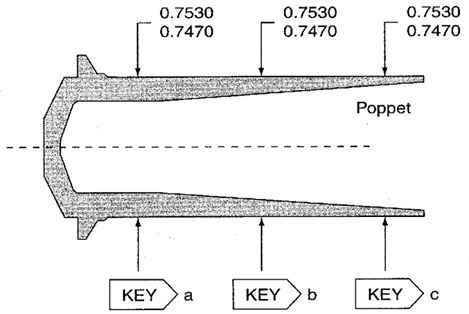Figure 1. Three OD key characteristics on a poppet.

## Case Description

A poppet is manufactured on a screw machine. Rejection rates due to inconsistent ODs have been unacceptably high. Therefore, uniformity of the OD is designated as a key characteristic. To check the uniformity, three OD measurements arc taken on each poppet at locations a, b, and c. Although the dimensions of the poppet could also be monitored using three separate Xbar-R charts—one for each dimension—quality assurance wants to monitor the diameter using only one chart. This is why the group Xbar-R chart is selected.

### Bring SPC Charts Up to Speed

This example provides a deep dive into the manual calculations behind the group Xbar-R chart. InfinityQS® solutions—ProFicient™ and Enact®—automate chart creation and help you optimize processes faster.

Sampling Strategy
Because the same characteristic is being measured at three different locations on the same part, a group Xbar-R chart is selected. Three poppets are measured every 15 minutes.

## Data Collection Sheet

Table 1. Data collection sheet for the group Xbar-R chart. MAX and MIN plot points for each group are displayed in bold.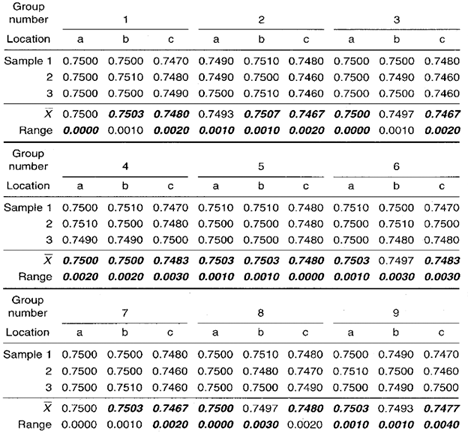## Group Xbar-R Chart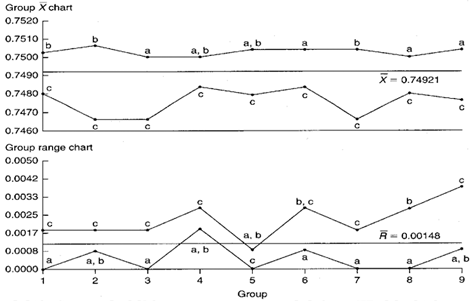Figure 2. Group Xbar-R charts representing three ODs on the same part.

## Chart Interpretation

Group range chart: Location c appears in the MAX position seven out of nine times. This strongly suggests that location c has the largest standard deviation. Location a appears eight out of nine times in the MIN position, therefore, location a most likely has the smallest standard deviation. The value of location b’s standard deviation falls somewhere between the value of the standard deviation of locations a and c.
Note: The centerline on the group range chart is the average of all 27 ranges found in Table 1.

Group Xbar chart: Locations a and b are in the MAX position six times and five times respectively. This sharing of the MAX position means that the average diameters of a and b behave similarly and they are always larger than location c, which appears nine out of nine times in the MIN position.
Note: The centerline on the group Xbar chart is the average of all 27 Xbar values found in Table 1.

## Recommendations

• These charts illustrate the lack of uniformity in the popper CD. The first recommendation is to change the process so that location c’s diameter increases enough to be in line with the size of the diameters at locations a and b. This might be done by reworking the cam or changing the program on the screw machine.
• The large amount of variation at location c should also be addressed. To do this, operators might try a different way of positioning the work piece material in the holding fixture or find a different way to machine the dimension at location c.

## Estimating the Process Average

Process average estimates should be performed separately for each characteristic or location on the group chart (see Calculation 1).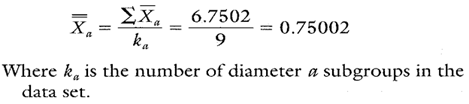Calculation 1. Estimate of the process average for location a.

## Estimating Sigma

Estimates of sigma are also calculated separately for each characteristic or location on the group chart. Continuing with location a, see Calculations 2 and 3.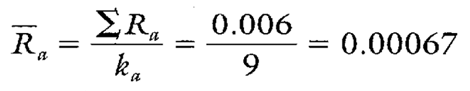Calculation 2. Calculation of R for location a.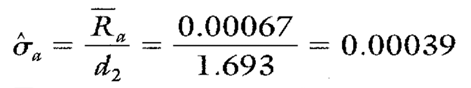Calculation 3. Estimated standard deviation for location a.
Note: To ensure reliable estimates, k needs to be at least 20. In this example, k is only nine. Therefore, these estimates and the ones found in Table 2 are shown only for illustration purposes.

## Calculating Process Capability and Performance Ratios

Calculations 4, 5, and 6 show the process capability and performance calculations for location a.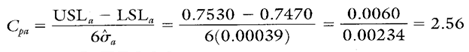Calculation 4. Cp calculation for location a.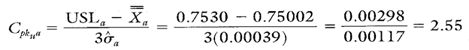Calculation 5. Cpk upper calculation for location a.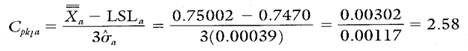Calculation 6. Cpk lower calculation for location a.

• Multiple characteristics can be tracked on one chart.
• Pinpoints the characteristics that are most in need of attention.
• Separates variation due to changes in the average from variation due to changes in the standard deviation.

• No visibility of the characteristics that fall between the MAX and MIN plot points
• Cannot detect certain out-of-control conditions because the group charts described here have no control limits

The process capability and performance calculations for locations b and c are shown in Table 2.

Table 2. Additional summary statistics and process capability and performance ratios.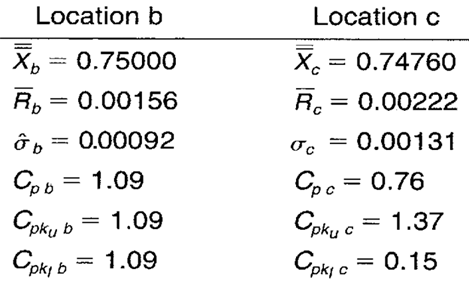FOOTNOTE:
1 Wise, Stephen A. and Douglas C. Fair. Innovative Control Charting: Practical SPC Solutions for Today’s Manufacturing Environment. Milwaukee, WI: ASQ Quality Press.

### How to Reduce the Cost and Time of Analyzing Quality Data

Are your manufacturing quality professionals spending numerous hours every week converting statistical process control (SPC) data for analysis? Your quality solution should make that analysis instant and automatic.

Learn what a difference a modern quality platform makes.

### Take the first step from quality to excellence

Take the Next Steps